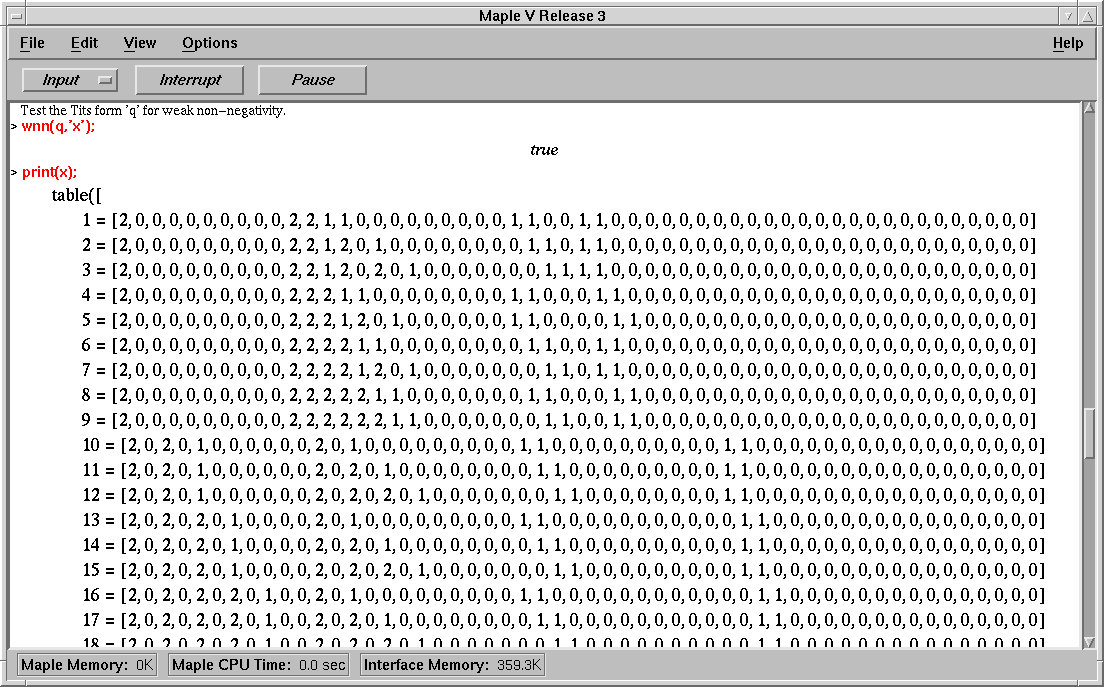### The function 'wnn'

The CREP function 'wnn' is used to test whether a unit form q - such as the Tits form in our example - is weakly non-negative, ie whether for any vector x of non negative integers the value of x under q is non-negative. If such is the case - as it turns out in our example - the (optional) second argument of the function is used to return a set of critical vectors for q. That is, a set of minimal vectors on which the unit form q is zero. In our example there being many such vectors, we display only some of them.The non negativity of the Tits form of an algebra is related to the representation type of that algebra in the following way: The algebra is known to be wild, in case the Tits form is not weakly non-negative, the reverse being in general an open question. So for the user in our example there still remains some work to be done, in order to decide about the representation type of the algebra considered.

Back to the worksheet of the example session.